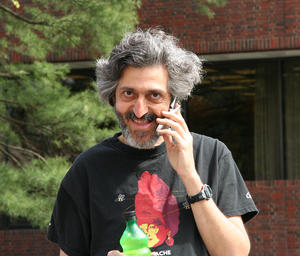# BARC-QMATH talk by Avi Wigderson

Title:
Optimization, Complexity and Math (through the lens of one problem and one algorithm)Abstract:
I will first introduce and motivate the main characters in this plot:

- Singularity of symbolic matrices: a basic problem in arithmetic complexity.

- Alternating Minimization: a basic heuristic in non-convex optimization.

I will then explain how variants of this algorithm are applied to variants of this problem, how these are analyzed, and how the analysis gives rise to problems in and connections between a surprisingly diverse set of mathematical areas. These include  quantum information theory, non-commutative algebra, analysis and invariant theory. Time permitting, I will discuss challenges this work raises in invariant theory and non-convex optimization.

No special background will be assumed.

Avi Wigderson will visit BARC and the Math Department May 14-15.
On May 15 he will be giving the Harald Bohr Lecture at the Math Department: https://www.math.ku.dk/english/calendar/events/hbl-wigderson/

BIO
Avi Wigderson
is an Israeli mathematician and computer scientist. He is professor of mathematics at the Institute for Advanced Study in Princeton. His research interests include complexity theory, parallel algorithms, graph theory, cryptography, distributed computing, and neural networks.
Wigderson received the Nevanlinna Prize in 1994 for his work on computational complexity. Along with Omer Reingold and Salil Vadhan he won the 2009 Gödel Prize for work on the zig-zag product of graphs, a method of combining smaller graphs to produce larger ones used in the construction of expander graphs. He was elected to the National Academy of Sciences in 2013. He was elected as an ACM Fellow in 2018 for "contributions to theoretical computer science and mathematics". In 2019, Wigderson was awarded the Knuth Prize for his contributions to "the foundations of computer science in areas including randomized computation, cryptography, circuit complexity, proof complexity, parallel computation, and our understanding of fundamental graph properties".